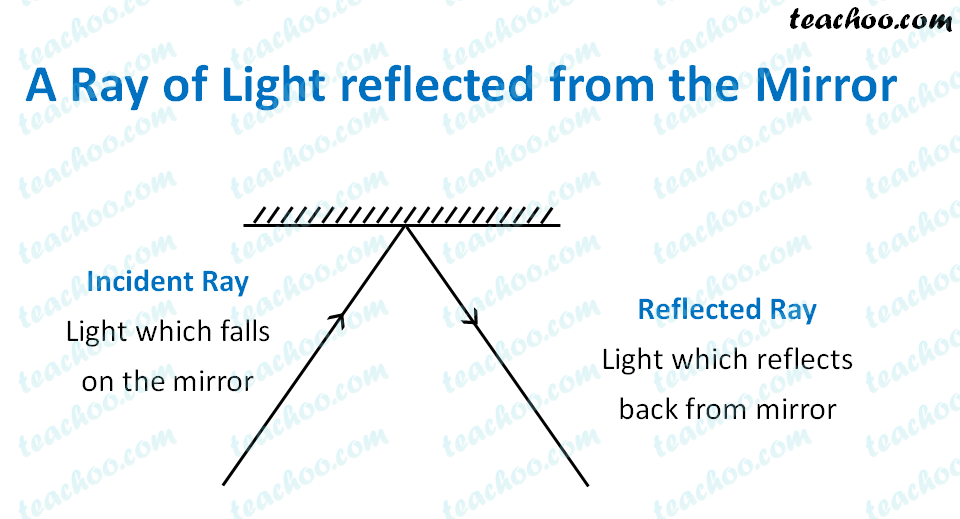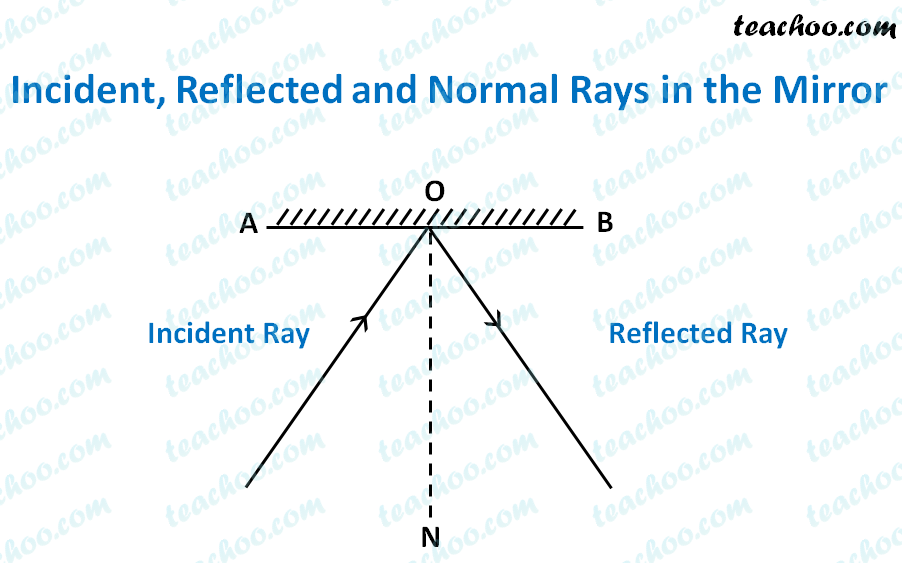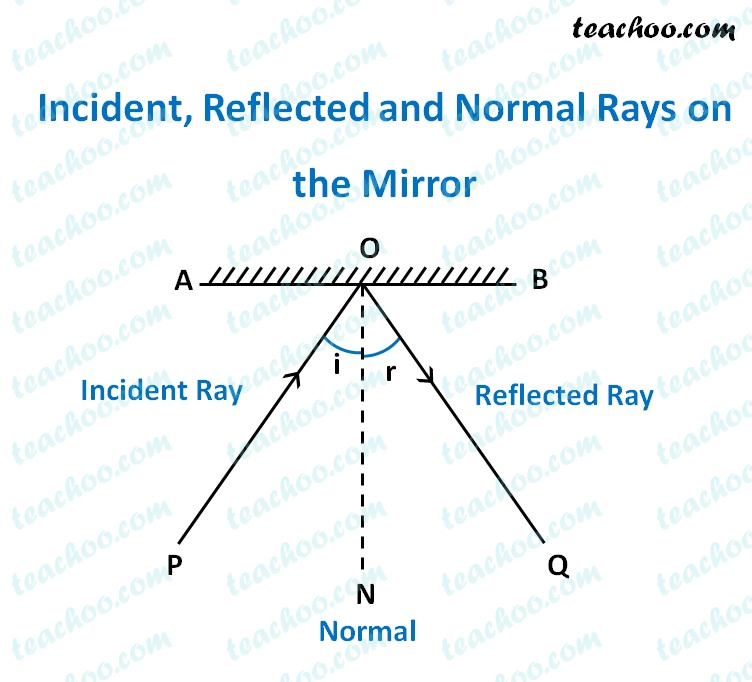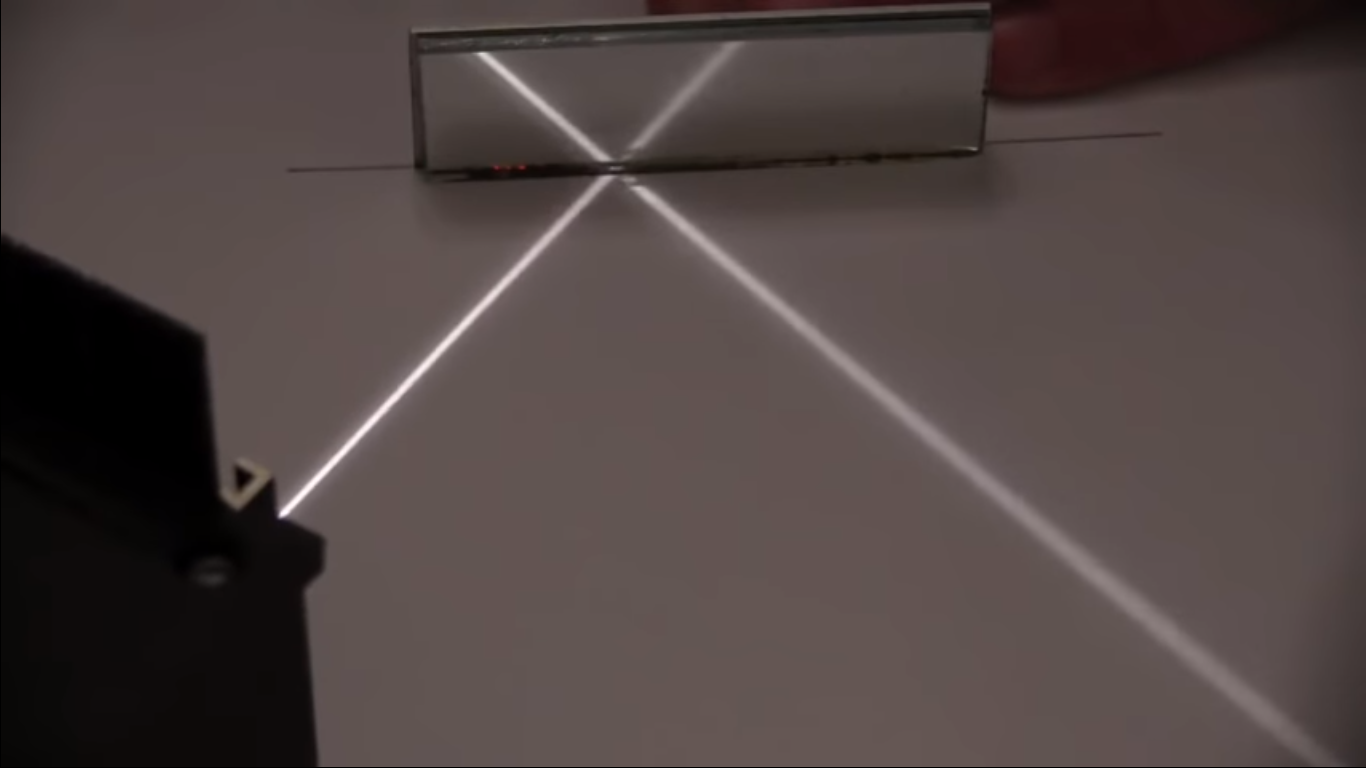Concepts

Class 8
Chapter 16 Class 8 - Light

## What happens when a light ray strikes a mirror?

When a ray of light strikes a mirror,it is reflected in a different direction## What is Incident Ray and Reflected Ray?

The light ray which strikes a surface is called Incident Ray

The light ray which comes back from surface after reflection is called Reflected Ray

## What is Normal

It is a line which is perpendicular to mirror at point of incidence

## How to Draw Normal

Let Mirror be denoted by AB

Let Point where incident ray strikes mirror be O

We draw a line ON which is perpendicular to mirror AB at point O

This ON is called Normal Ray## What is the Angle of Incidence and Reflection?

Angle between Normal and Incident Ray is called Angle of Incidence

Let it be denoted by i

Angle between Normal and Reflected Ray is called Angle of Reflection

Let it be denoted by r

Note that

Angle of Incidence is always equal to the Angle of Reflection

In our case,

Angle i = Angle r## Laws of Reflection

There are 2 laws of Reflection

1. The Incident ray, Reflected ray and the Normal, all lie in the same plane
2. The Angle of Incidence is always equal to Angle of Reflection

So, in our figure above,

Incident ray OP, reflected ray OQ and Normal ray ON all lie on the same plane

And,

Angle of incidence = Angle of Reflection

Check the figure belowIn this figure, the incident ray, reflected ray and normal all lie on the table. So, they all lie on the same plane.

And, Angle of Incidence = Angle of Reflection.

Check out the video below for the full experiment

Get live Maths 1-on-1 Classs - Class 6 to 12